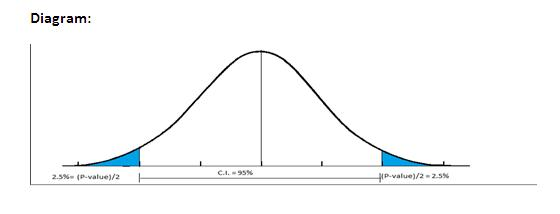# P Value Significance Probability

## What is P Value Significance Probability?

The P value is the probability value for a statistical hypothesis testing and is the probability of getting the value of the test statistic beyond the extreme positions for which the null hypothesis (H0) is true.

The significance level is the probability for which the null hypothesis is to be rejected and is the probability beyond the extreme points for the null hypothesis and hence, equal to the p value.

Example: For example, for a 95% confidence level for a hypothesis test, the significance level is 5%. If the p value for the test comes out to be less than 5%, that is the significance level, we can reject the null hypothesis.In other words, for rejected null hypothesis of 95% confidence interval, we can state p<0.05.

Therefore, smaller the p value, more the chances of the null hypothesis being false.This article has been researched & authored by the Business Concepts Team. It has been reviewed & published by the MBA Skool Team. The content on MBA Skool has been created for educational & academic purpose only.

Browse the definition and meaning of more similar terms. The Management Dictionary covers over 2000 business concepts from 6 categories.

Search & Explore : Business Concepts